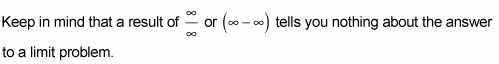##### Calculus II For DummiesHorizontal asymptotes and limits at infinity always go hand in hand. You can’t have one without the other. If you’ve got a rational function like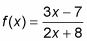determining the limit at infinity or negative infinity is the same as finding the location of the horizontal asymptote.

Here’s what you do. First, note the degree of the numerator (that’s the highest power of x in the numerator) and the degree of the denominator. Now, you've got three cases:

• If the degree of the numerator is greater than the degree of the denominator, for example: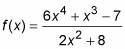there’s no horizontal asymptote and the limit of the function as x approaches infinity (or negative infinity) does not exist.

• If the degree of the denominator is greater than the degree of the numerator, for example: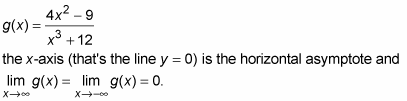• If the degrees of the numerator and denominator are equal, take the coefficient of the highest power of x in the numerator and divide it by the coefficient of the highest power of x in the denominator. That quotient gives you the answer to the limit problem and the heightof the asymptote.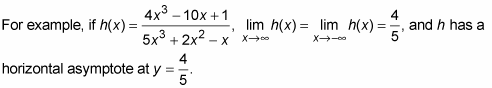Keep in mind that substitution often doesn’t work for problems that deal with limits at infinity.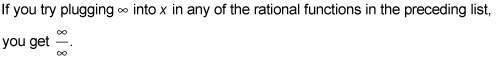But that does not equal 1. (Nor, by the way, does infinity minus infinity equal zero.)